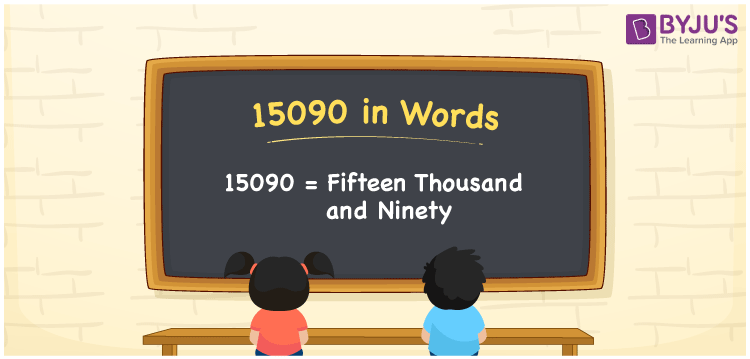# 15090 in Words

We can write 15090 in words as Fifteen thousand and ninety. Thus, the number name or word form of 15090 is Fifteen thousand and ninety. This word form of 15090 is used to express or communicate the number. For example, when you receive Rs. 15090 as a special allowance, then you can say, “I received Fifteen thousand and ninety rupees as the special allowance”. In this article, you will learn how to write the cardinal number 15090 in word form using a place value chart.

 15090 in words Fifteen thousand and ninety Fifteen thousand and ninety in Numbers 15090

## 15090 in English Words

Generally, we use the English alphabet to write numbers in words. Thus, we spell 15090 in English words as “Fifteen thousand and ninety”.## How to Write 15090 in Words?

The below table depicts the place value chart for the numeral 15090. Using this table, we can convert 15090 into words.

 Ten thousand Thousands Hundreds Tens Ones 1 5 0 9 0

Here, ones = 0, tens = 9, hundreds = 0, thousands = 5, ten thousand = 1

Let us expand these numbers, as given below.

1 × Ten thousand + 5 × Thousand + 0 × Hundred + 9 × Ten + 0 × One

= 1 × 10000 + 5 × 1000 + 0 × 100 + 9 × 10 + 0 × 1

= 10000 + 5000 + 90

= Ten Thousand + Five thousand + Ninety

= Fifteen thousand + Ninety

= Fifteen thousand and ninety

Therefore, 15090 in words = Fifteen thousand and ninety.

15090 is a natural number that is the successor of 15089 and predecessor of 15091.

15090 in words – Fifteen thousand and ninety

Is 15090 an odd number? – No

Is 15090 an even number? – Yes

Is 15090 a perfect square number? – No

Is 15090 a perfect cube number? – No

Is 15090 a prime number? – No

Is 15090 a composite number? – Yes

## Frequently Asked Questions on 15090 in Words

Q1

### How do you write 15090 in English words?

We can write the number 15090 in English words as Fifteen thousand and ninety.
Q2

### How do you write Rs. 15090 in words on a cheque?

On a cheque, we generally write Rs. 15090 in words as “Fifteen thousand and ninety rupees only”.
Q3

### What is 15090 in words?

Fifteen thousand and ninety is known as 15090 in words.